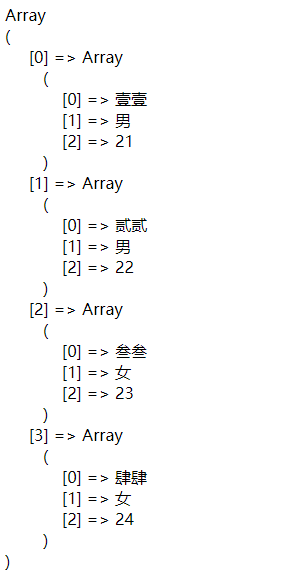# PHP中mysqli_select_db与mysqli_query函数的用法举例

PHP通过mysqli_connect() 函数可以去连接MySQL数据库，其中该函数有一个参数是对应数据库的名称，这个参数是可选参数，是可以省略的。如果将这个参数省略的话还需要在后面指定一个默认的数据库，PHP中就可以通过mysqli_select_db() 函数来指定一个默认数据库。一起来看看这个函数的用法。

mysqli_select_db()函数

``````mysqli::select_db(string \$dbname)
``````

``````mysqli_select_db(mysqli \$link, string \$dbname)
``````

\$dbname 还是表示为指定的数据库名称，

``````    \$host     = 'localhost';
\$dbname   = 'test';
if(\$mysql -> connect_errno){
die('数据库连接失败：'.\$mysql->connect_errno);
}else{
\$mysql -> select_db(\$dbname);                  // 选择名为 test 的数据库
\$sql    = 'select name,sex,age from user';     // SQL 语句
\$result = \$mysql -> query(\$sql);               // 执行上面的 SQL 语句
\$data   = \$result -> fetch_all();
\$mysql -> close();
}
echo '<pre>';
print_r(\$data);
``````

``````    \$host     = 'localhost';
\$dbname   = 'test';
mysqli_select_db(\$link, \$dbname);           // 选择名为 test 的数据库
\$sql    = 'select name,sex,age from user';  // SQL 语句
\$result = mysqli_query(\$link, \$sql);        // 执行 SQL 语句，并返回结果
\$data   = mysqli_fetch_all(\$result);        // 从结果集中获取所有数据
}else{
echo '数据库连接失败！';
}
echo '<pre>';
print_r(\$data);
``````

mysqli_query() 函数

``````mysqli::query( string \$query [, int \$resultmode = MYSQLI_STORE_RESULT ] )
``````

\$query 表示要执行的 SQL 语句；

\$resultmode 是可选参数，用来修改函数的行为。

``````mysqli_query( mysqli \$link , string \$query [, int \$resultmode = MYSQLI_STORE_RESULT ] )
``````

\$query 表示要执行的 SQL 语句；

\$resultmode 是可选参数，用来修改函数的行为。

``````mysql> select * from user;
+----+--------+------+------+
| id | name | age | sex |
+----+--------+------+------+
| 1 | 壹壹 | 21 | 男 |
| 2 | 贰贰 | 22 | 男 |
| 3 | 叁叁 | 23 | 女 |
| 4 | 肆肆 | 24 | 女 |
+----+--------+------+------+
4 rows in set (0.00 sec)
``````

``````    \$host     = 'localhost';
\$dbname   = 'test';
if(\$mysql -> connect_errno){
die('数据库连接失败：'.\$mysql->connect_errno);
}else{
\$mysql -> set_charset('UTF-8');             // 设置数据库字符集
\$sql = 'select name,sex,age from user';     // SQL 语句
\$result = \$mysql -> query(\$sql);            // 执行上面的 SQL 语句
\$data = \$result -> fetch_all();
\$mysql -> close();
}
echo '<pre>';
print_r(\$data);
?>
````````````    \$host     = 'localhost';
\$dbname   = 'test';
\$sql    = 'select name,sex,age from user';  // SQL 语句
\$result = mysqli_query(\$link, \$sql);        // 执行 SQL 语句，并返回结果
\$data   = mysqli_fetch_all(\$result);        // 从结果集中获取所有数据